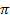## Two-sided p-values

Now, sample proportions that are much larger than 0.74 OR much smaller than 0.74 will be considered evidence against the null hypothesis and in favor of the alternative hypothesis. In other words, we will look for values at least as extreme as the observed proportion in either direction.

In the actual study, Dr. Güntürkün observed 80 of the 124 couples in his sample turn to the right.

(c) Calculate the observed statistic. What symbol can we use to represent this value?

• Use the One Proportion applet below to simulate a null distribution for sample proportions assuming= 0.74 and n = 124.

(d) Is your sample proportion in the tail of this distribution?

• Now check the Two-sided box. Include a screen capture of your output, showing the p-value, in your lab report.

(e) What values does the applet consider as or more extreme as the observed sample proportion?

(f) Based on this p-value, would you say there is convincing evidence thatdiffers from 0.74? Explain your reasoning.